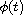Electron. J. Diff. Equ., Vol. 2015 (2015), No. 11, pp. 1-20.

### Quasi-periodic solutions of nonlinear beam equations with quintic quasi-periodic nonlinearities Qiuju Tuo, Jianguo Si

Abstract:
In this article, we consider the one-dimensional nonlinear beam equations with quasi-periodic quintic nonlinearitiesunder periodic boundary conditions, where B is a positive constant,is a small positive parameter,is a real analytic quasi-periodic function in t with frequency vector. It is proved that the above equation admits many quasi-periodic solutions by KAM theory and partial Birkhoff normal form.

Submitted October 24, 2014. Published January 7, 2015.
Math Subject Classifications: 35L05, 37K50, 58E05.
Key Words: Infinite dimensional Hamiltonian systems; KAM theory; beam equations; quasi-periodic solutions; invariant torus.

Show me the PDF file (330 KB), TEX file, and other files for this article.Qiuju Tuo School of Mathematics, Shandong University Jinan, Shandong 250100, China email: qiujutuo@163.com Jianguo Si School of Mathematics, Shandong University Jinan, Shandong 250100, China email: sijgmath@sdu.edu.cn